# Interval Notation

Interval notation is a method of writing down a set of numbers. Usually, this is used to describe a certain span or group of spans of numbers along a axis, such as an x-axis. However, this notation can be used to describe any group of numbers.

For example, consider the set of numbers that are all greater than 5. If we were to write an inequality for this set, letting x be any number in the group, we would say: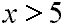This same set could be described in another type of notation called interval notation. In that notation the group of numbers would be written as: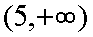Here is how to interpret this notation:

• The span of numbers included in the group is often imagined as being on a number line, usually the x-axis.
• The '(5' on the left means the set of numbers starts at the real number which is immediately to the right of 5 on the number line. It means you should imagine a number the tinniest bit greater than 5, and that is where the group of numbers begins. The parenthesis to the left of 5 is called a round bracket or an exclusive bracket. That is, 5 is excluded from the group, but the numbers directly to the right of 5 are included. Simply put, numbers greater than 5 are included.
• The group of numbers continues to include values greater than 5 all the way to a value which is infinitely greater than 5. That is, the set of numbers goes all the way to positive infinity. That is what the positive infinity symbol on the right means.
• Infinity symbols are always accompanied by round brackets.

Now consider the group of numbers that are equal to 5 or greater than 5. That group would be described by this inequality: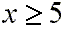In interval notation this set of numbers would look like this: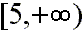This interval notation would be interpreted just like the interval above, except:

• The '[5' on the left means the set of numbers starts on the number line with 5. The square bracket to the left of 5 is called an inclusive bracket. That is, 5 is included within the group. Simply put, the number 5 and all numbers greater than 5 are included.

Now, what about numbers greater than 5 but less than 7? Expressed as an inequality this group would look like this: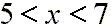This same group of numbers expressed with interval notation would look like this: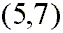Again:

• The round, exclusive brackets on the left and right mean 'up to but not including'.

And here is an inequality showing a group of numbers equal to or greater than 5 and less than 7: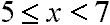Here is this group of numbers expressed with interval notation: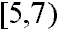Notice that there is a square, or inclusive, bracket on the left of this interval notation next to the 5. This means that this group of numbers starts at 5 and continues for values greater than 5. The round bracket on the right next to the 7 is, again, an exclusive bracket. This means that the numbers in this group have values up to but not including the 7.

Well, by now, hopefully interval notation is clear to you. Let us go through one last simple example. Consider the group of numbers equal to or greater than 5 and less than or equal to 7. An inequality for this set would look like this;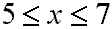Since both the 5 and the 7 are included in the group we will need inclusive, or square, brackets at each end of the interval notation. That notation looks like this: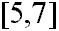Well, let us get just a bit more complicated. Using interval notation we will show the set of number that includes all real numbers except 5. First, stated as inequalities this group looks like this: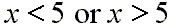The statement using the inequalities above joined by the word or means that x is a number in the set we just described, and that you will find that number somewhere less than 5 or somewhere greater than 5 on the number line.

In interval notation a logically equivalent statement does not use the word or, but rather a symbol for what is called the union of two groups of numbers. The symbol for union coincidentally looks like a U, the first letter of union. However, it is really not a letter of the alphabet. Here is what the union symbol looks like: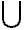So, the group of numbers that includes all values less than 5 and all values greater than 5, but does not include 5 itself, expressed as interval notation looks like this: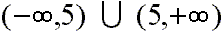Let us consider one last set of numbers. We will consider a group of numbers containing all numbers less than or equal to 5 and also those numbers that are greater than 7 but less than or equal to 12. Using inequalities this group of numbers could be notated like this: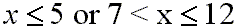And using interval notation as described throughout this material this group would look like this: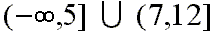We would interpret this interval notation as representing the total group of numbers as the union of two other groups. The first would start at negative infinity and proceed toward the right down the number line up to and including 5. The second would start just to the right of 7, but not including 7, and continue to the right down the number line up to and including 12. The total set of numbers would be all those in the first group along with all of those in the second, and this would be the same total group of numbers which we considered in the above inequality where we first introduced this last example.

So, we see that interval notation is useful for stating the members of groups of numbers. It is often used to state the set of numbers which make up the domain and range of a function.

Custom Search